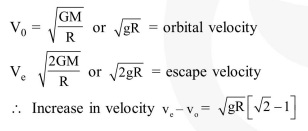# A satellite is reolving in a

Question:

A satellite is reolving in a circular orbit at a height ' $h$ ' from the earth's surface (radius of earth $\mathrm{R} ; \mathrm{h}<<\mathrm{R}$ ). The minimum increase in its orbital velocity required, so that the satellite could escape from the earth's gravitational field, is close to : (Neglect the effect of atmosphere).

1. $\sqrt{g R}(\sqrt{2}-1)$

2. $\sqrt{2 g R}$

3. $\sqrt{\mathrm{gR}}$

4. $\sqrt{g R / 2}$

Correct Option: 1

Solution: